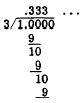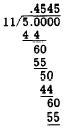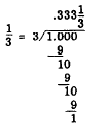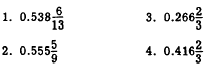Custom SearchNonterminating Decimals  As stated previously, if the denominator of a common fraction contains some prime factor other than 2 or 5, the fraction cannot be converted completely to a decimal. When such fractions are converted according to the foregoing rule, the decimal resulting will never terminate. Consider the fraction 1/3. Applying the rule, we haveThe division will continue indefinitely. Any common fraction that cannot be converted exactly yields a decimal that will never terminate and in which the digits sooner or later recur. In the previous example, the recurring digit was 3. In the fraction 5/11, we haveThe recurring digits are 4 and 5. When a common fraction generates such a repeating decimal, it becomes necessary to arbitrarily select a point at which to cease the repetition. This may be done in two ways. We may write the decimal fraction by rounding off at the desired point. For example, to round off . the decimal generated by 1/3 to hundredths, we carry the division to thousandths, see that this. figure is less than 5, and drop it. Thus, 1/3 rounded to hundredth6 is 0.33. The other method is to carry the division to the desired number of decimal places and carry the remaining incomplete division as a common fraction-that is, we write the result of a complex decimal. For example, 1/3 carried to thousandths would bePractice problems. Change the following common fraction6 to decimals with three places and carry the incomplete division as a common fraction:Answers:OPERATION WITH DECIMALS In the study of addition of whole numbers, it was established that units must be added to units, tens to tens, hundred6 to hundreds, etc. For convenience, in adding several numbers, units were written under units, ten6 under tens, etc. The addition of decimals is accomplished in the same manner. ADDITION In adding decimals, tenths are written under tenths, hundredth6 under hundredths, etc. When this is done, the decimal points fall in a straight line. The addition is the same as in adding whole numbers. Consider the following example:Adding the first column on the right gives 17 hundredths or 1 tenth and 7 hundredths. As with whole numbers, we write the 7 under the hundredths column and add the 1 tenth in the tenths column-that is, the column of the next higher order. The sum of the tenths column is 15 tenths or 1 unit and 5 tenths. The 5 is written under the tenths column and the 1 is added in the units column. It is evident that if the decimal points are kept in a straight line-that is, if the place values are kept in the proper columns-addition with decimals may be accomplished in the ordinary manner of addition of whole numbers. It should also be noted that the decimal point of the sum falls directly under the decimal points of the addends. SUBTRACTION Subtraction of decimals likewise involves no new principles. Notice that the place values of the subtrahend in the following example are fixed directly under the corresponding place values in the minuend. Notice also that this causes the decimal points to be aligned and that the figures in the difference (answer) also retain the correct columnar alignment.We subtract column by column, as with whole numbers, beginning at the right. Practice problems. Add or Subtract as indicated: 1. 12.3 + 2.13 + 4 + 1.234 2. 0.5 + 0.04 + 12.001 + 10 3. 237.5 - 217.9 4. 9.04 - 7.156 Answers: 1. 19.664 2. 22.541 3. 19.6 4. 1.184 MULTIPLICATION Multiplication of a decimal by a whole number may be explained by expressing the decimal as a fraction. EXAMPLE: Multiply 6.12 by 4. SOLUTION:When we perform the multiplication keeping the decimal form, we haveBy common sense, it is apparent that the whole number 4 times the whole number 6, with some fraction, will yield a number in the neighborhood of 24. Hence, the placing of the decimal point is reasonable. An examination of several examples will reveal that the product of a decimal and a whole number has the same number of decimal places as the factor containing the decimal. Zeros, if any, at the end of the decimal should be rejected.Integrated Publishing, Inc. - A (SDVOSB) Service Disabled Veteran Owned Small Business